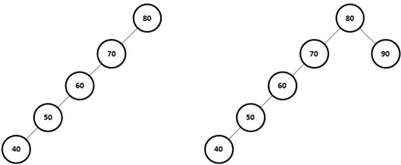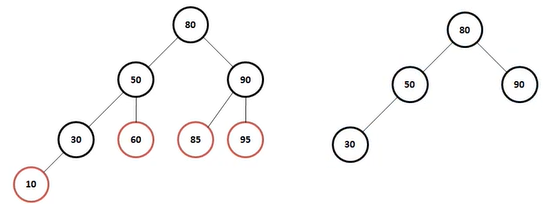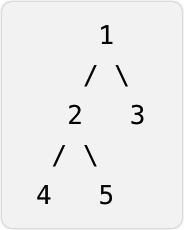java实现简单二叉树

+关注继续查看

一、树的定义

?（1）每个结点有零个或多个子结点

（2）没有父节点的结点称为根节点

（3）每一个非根结点有且只有一个父节点

（4）除了根结点外，每个子结点可以分为多个不相交的子树。

二、二叉树

1、二叉树的定义2、二叉树的性质

?

3、性质4的证明

三、满二叉树、完全二叉树和二叉查找树

1、满二叉树

?2、完全二叉树3、二叉查找树（1）若任意结点的左子树不空，则左子树上所有结点的值均小于它的根结点的值。

（2）任意结点的右子树不空，则右子树上所有结点的值均大于它的根结点的值。

（3）任意结点的左、右子树也分别为二叉查找树。

（4）没有键值相等的结点。

import java.util.ArrayList;
import java.util.List;

public class bintree {
public bintree left;
public bintree right;
public bintree root;
//    数据域
private Object data;
//    存节点
public List<bintree> datas;

public bintree(bintree left, bintree right, Object data){
this.left=left;
this.right=right;
this.data=data;
}
//    将初始的左右孩子值为空
public bintree(Object data){
this(null,null,data);
}

public bintree() {

}

public void creat(Object[] objs){
datas=new ArrayList<bintree>();
//        将一个数组的值依次转换为Node节点
for(Object o:objs){
}
//        第一个数为根节点
root=datas.get(0);
//        建立二叉树
for (int i = 0; i <objs.length/2; i++) {
//            左孩子
datas.get(i).left=datas.get(i*2+1);
//            右孩子
if(i*2+2<datas.size()){//避免偶数的时候 下标越界
datas.get(i).right=datas.get(i*2+2);
}

}

}
//先序遍历
public void preorder(bintree root){
if(root!=null){
System.out.println(root.data);
preorder(root.left);
preorder(root.right);
}

}
//中序遍历
public void inorder(bintree root){
if(root!=null){
inorder(root.left);
System.out.println(root.data);
inorder(root.right);
}

}
//    后序遍历
public void afterorder(bintree root){
if(root!=null){
System.out.println(root.data);
afterorder(root.left);
afterorder(root.right);
}

}

public static void main(String[] args) {
bintree bintree=new bintree();
Object []a={2,4,5,7,1,6,12,32,51,22};
bintree.creat(a);
bintree.preorder(bintree.root);
}
}

import java.util.Scanner;

public class btree {
private btree left,right;
private char data;
public btree creat(String des){
Scanner scanner=new Scanner(System.in);
System.out.println("des:"+des);
String str=scanner.next();
if(str.charAt(0)<'a')return null;
btree root=new btree();
root.data=str.charAt(0);
root.left=creat(str.charAt(0)+"左子树");
root.right=creat(str.charAt(0)+"右子树");
return root;
}
public void midprint(btree btree){
//        中序遍历
if(btree!=null){
midprint(btree.left);
System.out.print(btree.data+"  ");
midprint(btree.right);
}
}
public void firprint(btree btree){
//        先序遍历
if(btree!=null){
System.out.print(btree.data+" ");
firprint(btree.left);
firprint(btree.right);
}
}
public void lastprint(btree btree){
//        后序遍历
if(btree!=null){
lastprint(btree.left);
lastprint(btree.right);
System.out.print(btree.data+"  ");
}
}

public static void main(String[] args) {
btree tree = new btree();
btree newtree=tree.creat("根节点");
tree.firprint(newtree);
System.out.println();
tree.midprint(newtree);
System.out.println();
tree.lastprint(newtree);
}
}

des:根节点

a

des:a左子树

e

des:e左子树

c

des:c左子树

1

des:c右子树

1

des:e右子树

b

des:b左子树

1

des:b右子树

1

des:a右子树

d

des:d左子树

f

des:f左子树

1

des:f右子树

1

des:d右子树

1

a e c b d f ? ? ? ?先序

c ?e ?b ?a ?f ?d ? 中序

c ?b ?e ?f ?d ?a ? 后序? ??前序遍历：ABCDEFGHK

????中序遍历：BDCAEHGKF

????后序遍历：DCBHKGFEA9529 01155 01100 0【leetcode刷题】27.二叉树的直径——Java版
?欢迎订阅《leetcode》专栏，每日一题，每天进步? 可以将二叉树的直径转换为：二叉树的每个节点的左右子树的高度和的最大值。 ——leetcode此题热评
11 013222 0JavaScript实现排序二叉树的基本操作
1383 011540 06792 0javascript二叉树基本功能实现

832 06932 0
121

0

《2021云上架构与运维峰会演讲合集》

《零基础CSS入门教程》

《零基础HTML入门教程》